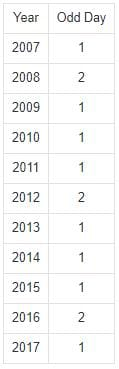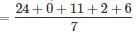Test: Calendar 1

# Test: Calendar 1

Test Description

## 20 Questions MCQ Test UPSC Prelims Paper 2 CSAT - Quant, Verbal & Decision Making | Test: Calendar 1

Test: Calendar 1 for Quant 2022 is part of UPSC Prelims Paper 2 CSAT - Quant, Verbal & Decision Making preparation. The Test: Calendar 1 questions and answers have been prepared according to the Quant exam syllabus.The Test: Calendar 1 MCQs are made for Quant 2022 Exam. Find important definitions, questions, notes, meanings, examples, exercises, MCQs and online tests for Test: Calendar 1 below.
Solutions of Test: Calendar 1 questions in English are available as part of our UPSC Prelims Paper 2 CSAT - Quant, Verbal & Decision Making for Quant & Test: Calendar 1 solutions in Hindi for UPSC Prelims Paper 2 CSAT - Quant, Verbal & Decision Making course. Download more important topics, notes, lectures and mock test series for Quant Exam by signing up for free. Attempt Test: Calendar 1 | 20 questions in 25 minutes | Mock test for Quant preparation | Free important questions MCQ to study UPSC Prelims Paper 2 CSAT - Quant, Verbal & Decision Making for Quant Exam | Download free PDF with solutions
 1 Crore+ students have signed up on EduRev. Have you?
Test: Calendar 1 - Question 1

### Today is Monday. After 61 days, it will be:

Detailed Solution for Test: Calendar 1 - Question 1

Each day of the week is repeated after 7 days.
So, after 63 days, it will be Monday.
∴ After 61 days, it will be Saturday.

Test: Calendar 1 - Question 2

### January 1st 1992 was a Wednesday. what day of the week was January 1, 1993?

Detailed Solution for Test: Calendar 1 - Question 2

1992 being a leap year it had two odd days show the first day of the year 1993 was today beyond so Wednesday i.e it was Friday.

Test: Calendar 1 - Question 3

### It was Sunday on Jan 1, 2006. What was the day of the week Jan 1, 2010?

Detailed Solution for Test: Calendar 1 - Question 3

On 31st December, 2005 it was Saturday.
Number of odd days from the year 2006 to the year 2009 = (1 + 1 + 2 + 1) = 5 days.
∴ On 31st December 2009, it was Thursday.
Thus, on 1st Jan, 2010 it is Friday.

Test: Calendar 1 - Question 4

What was the day of the week on 28th May, 2006?

Detailed Solution for Test: Calendar 1 - Question 4

28 May, 2006 i.e. 2005 years and Period from 1.1.2006 to 28.5.2006

Odd days in 1600 years = 0
Odd days in 400 years = 0

5 years = 4 ordinary years + 1 leap year = (4 x 1) + (1 x 2) = 6 odd days

Jan (31) + Feb (28) + March (31) + April (30) + May (28) = 148 days
∴ 148 days = 21 weeks + 1 day = 1 odd day
Total number of odd days = 0 + 0 + 6 + 1 = 7 = 0 odd day.

Thus, the given day is Sunday.

Test: Calendar 1 - Question 5

What was the day of the week on 17th June, 1998?

Detailed Solution for Test: Calendar 1 - Question 5

17th June, 1998 i.e.1997 years + Period from 1.1.1998 to 17.6.1998

Odd days in 1600 years = 0
Odd days in 300 years = 5 x 3 = 1

97 years = 24 leap years + 73 ordinary years.
Number of odd days in 97 years ( 24 x 2 + 73) = 121 = 2 odd days.

Jan (31) + Feb (28) + March (31) + April (30) + May (31) + June (17) = 168 days
Therefore 168 days = 24 weeks = 0 odd day.

Total number of odd days = (0 + 1 + 2 + 0) = 3.

Thus, the given day is Wednesday.

Test: Calendar 1 - Question 6

What will be the day of the week 15th August, 2010?

Detailed Solution for Test: Calendar 1 - Question 6

15th August, 2010 = (2009 years + Period 1.1.2010 to 15.8.2010)
Odd days in 1600 years = 0
Odd days in 400 years = 0
9 years = (2 leap years + 7 ordinary years) = (2 x 2 + 7 x 1) = 11 odd days ≡ 4 odd days.

Jan.     Feb.   March   April     Mayb   June     July       Aug.
(31   +   28   +   31   +   30   +   31   +   30   +   31   +   15) = 227 days

∴ 227 days = (32 weeks + 3 days) ≡ 3 odd days.
Total number of odd days = (0 + 0 + 4 + 3) = 7 ≡ 0 odd days.
Given day is Sunday.

Test: Calendar 1 - Question 7

If 6th March, 2005 is Monday, what was the day of the week on 6th March, 2004?

Detailed Solution for Test: Calendar 1 - Question 7

The year 2004 is a leap year. So, it has 2 odd days.
But, Feb 2004 not included because we are calculating from March 2004 to March 2005. So it has 1 odd day only.
∴ The day on 6th March, 2005 will be 1 day beyond the day on 6th March, 2004.
Given that, 6th March, 2005 is Monday.
∴ 6th March, 2004 is Sunday (1 day before to 6th March, 2005).

Test: Calendar 1 - Question 8

On what dates of April, 2001 did Wednesday fall?

Detailed Solution for Test: Calendar 1 - Question 8

We shall find the day on 1st April, 2001.
1st April, 2001 = (2000 years + Period from 1.1.2001 to 1.4.2001)
Odd days in 1600 years = 0
Odd days in 400 years = 0
Jan. Feb. March April
(31 + 28 + 31 + 1)     = 91 days ≡ 0 odd days.
Total number of odd days = (0 + 0 + 0) = 0
On 1st April, 2001 it was Sunday.
In April, 2001 Wednesday falls on 4th, 11th, 18th and 25th.

Test: Calendar 1 - Question 9

How many days are there in x weeks x days?

Detailed Solution for Test: Calendar 1 - Question 9

x weeks x days = (7x + x) days = 8x days.

Test: Calendar 1 - Question 10

On 8th Feb, 2005 it was Tuesday. What was the day of the week on 8th Feb, 2004?

Detailed Solution for Test: Calendar 1 - Question 10

The year 2004 is a leap year. It has 2 odd days.
∴ The day on 8th Feb, 2004 is 2 days before the day on 8th Feb, 2005.
Hence, this day is Sunday.

Test: Calendar 1 - Question 11

The calendar for the year 2007 will be the same for the year:

Detailed Solution for Test: Calendar 1 - Question 11

Count the number of odd days from the year 2007 onwards to get the sum equal to 0 odd day.Sum = 14 odd days ≡ 0 odd days.
∴ Calendar for the year 2018 will be the same as for the year 2007.

Test: Calendar 1 - Question 12

Which of the following is not a leap year?

Detailed Solution for Test: Calendar 1 - Question 12

The century divisible by 400 is a leap year.
∴ The year 700 is not a leap year.

Test: Calendar 1 - Question 13

On 8th Dec, 2007 Saturday falls. What day of the week was it on 8th Dec, 2006?

Detailed Solution for Test: Calendar 1 - Question 13

The year 2006 is an ordinary year. So, it has 1 odd day.
So, the day on 8th Dec, 2007 will be 1 day beyond the day on 8th Dec, 2006.
But, 8th Dec, 2007 is Saturday.
∴ 8th Dec, 2006 is Friday.

Test: Calendar 1 - Question 14

January 1, 2008 is Tuesday. What day of the week lies on Jan 1, 2009?

Detailed Solution for Test: Calendar 1 - Question 14

The year 2008 is a leap year. So, it has 2 odd days.
1st day of the year 2008 is Tuesday (Given)
So, 1st day of the year 2009 is 2 days beyond Tuesday.
Hence, it will be Thursday.

Test: Calendar 1 - Question 15

January 1, 2007 was Monday. What day of the week lies on Jan. 1, 2008?

Detailed Solution for Test: Calendar 1 - Question 15

The year 2007 is an ordinary year. So, it has 1 odd day.
1st day of the year 2007 was Monday.
1st day of the year 2008 will be 1 day beyond Monday.
Hence, it will be Tuesday.

Test: Calendar 1 - Question 16

What day of the week will 22 Apr 2222 be?

Detailed Solution for Test: Calendar 1 - Question 16

22 Apr 2222 i.e. 2221 years + period from 1-Jan-2222 to 22-Apr-2222

We know that number of odd days in 400 years = 0
Hence the number of odd days in 2000 years = 0 (Since 2000 is a perfect multiple of 400)

Number of odd days in the period 2001-2200 = Number of odd days in 200 years = 5 x 2 = 10 = 3

As we can reduce perfect multiples of 7 from odd days without affecting anything

Number of odd days in the period 2201-2221 = 16 normal years + 5 leap years = 16 x 1 + 5 x 2 = 16 + 10 = 26 = 5 odd days
Number of days from 1-Jan-2222 to 22 Apr 2222 = 31 (Jan) + 28 (Feb) + 31 (Mar) + 22(Apr) = 112

112 days = 0 odd day
Total number of odd days = (0 + 3 + 5 + 0) = 8 = 1 odd day = Monday

Hence 22 Apr 2222 is Monday

Test: Calendar 1 - Question 17

Today is Monday. After 61 days, it will be:

Detailed Solution for Test: Calendar 1 - Question 17

Each day of the week is repeated after 7 days. So, after 63 days, it will be on Monday. After 61 days, it will be on Saturday.

Test: Calendar 1 - Question 18

If today is Monday, what will be the day 350 days from now?

Detailed Solution for Test: Calendar 1 - Question 18

350 days, 350/7 = 50, no odd days, so it will be a Monday.

Test: Calendar 1 - Question 19

If today is Saturday, then what day of the week will be on the 338 days from today?

Detailed Solution for Test: Calendar 1 - Question 19

number of odd days in 338 days = 338 / 7 = 248 complete weeks + 2odd days. 2nd day after Saturday is Monday.

Test: Calendar 1 - Question 20

What was the day of the week on 24th July 2011?

Detailed Solution for Test: Calendar 1 - Question 20

Formula : (Date + Month code + No.of years + No.of leap year + Century code)/7= 43/7 =1
Thus, the day of the week on 24th July 2011will be Sunday.

## UPSC Prelims Paper 2 CSAT - Quant, Verbal & Decision Making

67 videos|50 docs|151 tests
 Use Code STAYHOME200 and get INR 200 additional OFF Use Coupon Code
Information about Test: Calendar 1 Page
In this test you can find the Exam questions for Test: Calendar 1 solved & explained in the simplest way possible. Besides giving Questions and answers for Test: Calendar 1, EduRev gives you an ample number of Online tests for practice

## UPSC Prelims Paper 2 CSAT - Quant, Verbal & Decision Making

67 videos|50 docs|151 tests